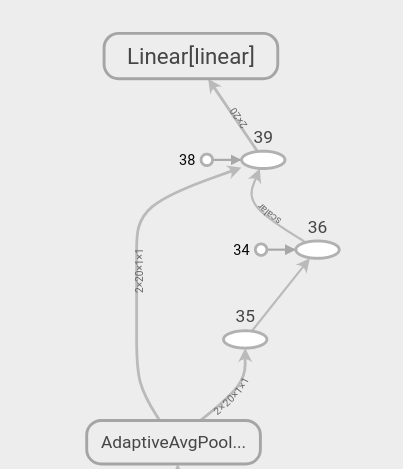# In tensorboard, how do I hide view() operations in a graph?

Hi, I added a graph and …
The graph shows operations of torch.Tensor.view()

``````def forward(self, x):
x = self.conv1(x)
x = self.conv2(x)
x = self.pull1(x)
x = self.conv3(x)
x = self.pull2(x)

x = x.view(x.size(0), -1)

x = self.linear(x)
``````It definitely annoys me… are there any ways to make a clean graph?
Thanks!